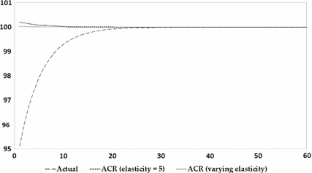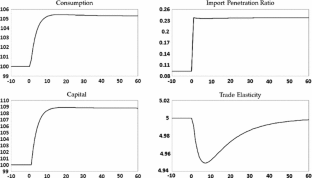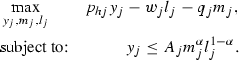# Capital accumulation and the welfare gains from trade

## Abstract

We measure the gains from a trade cost reduction in a model with dynamic accumulation of factors. We show that the tight link between import intensity and gains from trade that exists in static models breaks down along transition paths in dynamic models. When trade costs are reduced, the need to accumulate factors temporarily shifts spending from consumption to investment. Import intensity may rise or fall along the transition path, depending on the relative import intensity of consumption and investment. Calibrating the model to the US economy, we find that investment is more import intensive than consumption, so that import intensity is falling along the transition path even as consumption is rising. Therefore, while higher import intensity is associated with higher consumption when comparing steady states (as in static models), it is associated with lower consumption along a given transition path. We also consider the case of endogenous firm creation as another form of investment and factor accumulation, and again find a negative relationship between consumption and import intensity along the transition path.

This is a preview of subscription content, access via your institution.Fig. 1Fig. 2Fig. 3Fig. 4Fig. 5Fig. 6
1. 1.

That is, in the dynamic model, welfare is the permanent increase in consumption the household would need in the high trade cost equilibrium to be indifferent between that and the sequence of consumption she would receive with lower trade costs. Notice that this does take into account the transition.

2. 2.

In the appendix, we emphasize that channels that mimic the capital channel are present in models with multiple sectors, as in Section 3.4 of Costinot and Rodríguez-Clare (2014). Intuitively, trade implies cheaper intermediate goods, which reduces the marginal cost of production. We show that under a stark set of assumptions, there is an equivalence between that static model and a comparison of steady states in our dynamic model. In that case, the only margin that is different between the dynamic model and the static model with intermediate goods is the transition channel.

3. 3.

Although it captures the essential idea of hysteresis and aggregate consumption dynamics, this simplified model does not attempt to match the richer firm dynamics in Alessandria and Choi (2014).

4. 4.

This configuration of fixed costs guarantee that overshooting of consumption during the transition. Other configurations, such as ones where the fixed costs to operate and export are both sunk, may have different implications for transition dynamics. We focus on this case because our goal is to study a setting with consumption overshooting.

5. 5.

Notice that the fact that both factors are in fixed supply and production is constant returns to scale implies that factor prices will always move proportionally to one another. This is important for recovering the ACR result in this environment.

6. 6.

See Appendix “A.1” for the explicit characterization of the dynamic model used to derive this equation.

7. 7.

In the small open-economy case with a fixed interest rate, the transition lasts only one period.

8. 8.

Note that ACR assumes a single factor of production in fixed supply, while our static model has two factors of production. However, notice that if all modes of production use the same Cobb–Douglas production function, then all factors are used in fixed shares, and we can use cost minimization to define a composite factor and write an equivalent model that has that composite factor as a single factor of production. Therefore, the fact that we recover the ACR formula in this environment is not surprising.

9. 9.

The fact that computing the trade elasticities for consumption and investment goods separately would yield the welfare-relevant elasticity is because expenditure shares on consumption and investment are constant when $$\eta =1$$. In general, if expenditure shares change with trade costs that affects the trade elasticity, as shown in Brooks and Pujolas (2014).

10. 10.

Note that our choice of $$\tau ^H$$ is irrelevant, since the home bias parameters $$\mu$$ and $$\nu$$ will be used to match import shares.

11. 11.

Sector-level import intensity is measured as imports in that sector divided by the sum of final and intermediate uses in that sector.

12. 12.

In the input-output table, for consumption we use “Personal consumption expenditures” and for investment we use the sum of “Nonresidential private fixed investment in structures,” “Nonresidential private fixed investment in equipment,” “Nonresidential private fixed investment in intellectual property rights” and “Residential private fixed investment.” Importantly, we did not include inventories as investment, which likely includes durable consumption goods.

13. 13.

If the same industries that are more intensively used in investment are also the industries that are more intensively imported, then our exercise concludes that investment is more import intensive than consumption.

14. 14.

Import penetration is the ratio of imports to gross output. It is equal to $$1-\lambda _j$$.

15. 15.

Section 5.4 contains a discussion of how this overshooting dynamic arises.

16. 16.

In applying Eq. (16), we treat payment of fixed costs as $$E_{Ijt}$$.

17. 17.

The marginal entrant is the firm with productivity such that Eq. (38) holds with equality. That is, it is the lowest productivity operating firm from the high trade cost steady state.

18. 18.

The model is similar to the one presented in Section 3.4 of Costinot and Rodríguez-Clare (2014).

19. 19.

Under these assumptions, it can be shown that $$\nu$$, $$\mu$$, and $$\rho$$ are the same in both models. This can be proven using the characterizations in Appendices “A.1” and “A.3”.

## References

1. Adao, R., Costinot, A., Donaldson, D.: Nonparametric counterfactual predictions in neoclassical models of international trade. Am. Econ. Rev. 107(3), 633–689 (2017)

2. Alessandria, G., Choi, H.: Establishment heterogeneity, exporter dynamics, and the effects of trade liberalization. J. Int. Econ. 94(2), 207–223 (2014)

3. Alessandria, G., Choi, H., Ruhl, K.: Trade adjustment dynamics and the welfare gains from trade. NBER Working Paper 20663 (2014)

4. Alvarez, F., Buera, F., Lucas, R.: Idea flows, economic growth, and trade. NBER Working Paper 19667 (2013)

5. Anderson, J., Van Wincoop, E.: Trade costs. J. Econ. Lit. 42(3), 691–751 (2004)

6. Anderson, J.E., Larch, M., Yotov, Y.V.: Growth and trade with frictions: a structural estimation framework. NBER Working Paper 21377 (2015)

7. Arkolakis, C., Costinot, A., Donaldson, D., Rodriguez-Clare, A.: The elusive pro-competitive effects of trade. NBER Working Paper 21370 (2015)

8. Arkolakis, C., Costinot, A., Rodriguez-Clare, A.: New trade models, same old gains? Am. Econ. Rev. 102(1), 94–130 (2012)

9. Bajona, C., Kehoe, T.J.: Trade, growth, and convergence in a dynamic Heckscher–Ohlin model. Rev. Econ. Dyn. 13(3), 487–513 (2010)

10. Baldwin, R.E.: Measurable dynamic gains from trade. J. Polit. Econ. 100(1), 162–174 (1992)

11. Bernard, A., Jensen, J.B., Redding, S., Schott, P.: Firms in international trade. J. Econ. Perspect. 21(3), 105–130 (2007)

12. Boucekkine, R., Fabbri, G., Pintus, P.A.: Short-run pain, long-run gain: the conditional welfare gains from international financial integration. Econ. Theory. doi:10.1007/s00199-016-1019-7 (forthcoming) (2016)

13. Brooks, W., Pujolas, P.: Nonlinear gravity. McMaster Working Paper 2014–2015 (2014)

14. Chaney, T.: Distorted gravity: the intensive and extensive margins of international trade. Am. Econ. Rev. 98(4), 1707–1721 (2008)

15. Clementi, G., Palazzo, B.: Entry, exit, firm dynamics, and aggregate fluctuations. Am. Econ. J. Macroecon. 8(3), 1–41 (2016)

16. Costinot, A., Rodríguez-Clare, A.: Trade theory with numbers: quantifying the consequences of globalization. In: Gopinath, G., Helpman, E., Rogoff, K. (eds.) Handbook of International Economics, Chapter 4, vol. 4, pp. 197–261. Elsevier, Amsterdam (2014)

17. De Loecker, J., Warzynski, F.: Markups and firm level export status. Am. Econ. Rev 102(6), 2437–2471 (2012)

18. Edmond, C., Midrigan, V., Xu, D.: Competition, markups and the gains from international trade. Am. Econ. Rev. 105(10), 3183–3221 (2015)

19. Gourinchas, P.-O., Jeanne, O.: The elusive gains from international financial integration. Rev. Econ. Stud. 73(3), 715–741 (2006)

20. Havranek, T., Horvath, R., Irsova, Z., Rusnak, M.: Cross-country heterogeneity in intertemporal substitution. J. Int. Econ. 96(1), 100–118 (2015)

21. Holmes, T.J., Hsu, W.-T., Lee, S.: Allocative efficiency, mark-ups, and the welfare gains from trade. J. Int. Econ. 94(2), 195–206 (2015)

22. Kemp, M.C., Wong, K.-Y.: Gains from trade with overlapping generations. Econ. Theory 6(2), 283–303 (1995)

23. Ravikumar, B., Santacreu, A.M., Sposi, M.: Capital accumulation and the dynamic gains from trade. Working Paper (2017)

24. Rivera-Batiz, L.A., Romer, P.M.: Economic integration and endogenous growth. Q. J. Econ. 106(2), 531–555 (1991)

25. Ruhl, K.J.: The international elasticity puzzle. Working Paper (2008)

26. Young, A.: Learning by doing and the dynamic effects of international trade. Q. J. Econ. 106(2), 369–405 (1991)

Download references

## Author information

Authors

### Corresponding author

Correspondence to Pau S. Pujolas.

## Additional information

We are grateful to Tim Kehoe, Juan Carlos Conesa, two excellent anonymous referees and our editor, Costas Arkolakis.

## Appendices

### Appendix A: Model characterization

In this appendix, we characterize of equilibria for each of the models presented in Sect. 2 and the model from Sect. 5. We consider the symmetric country cases in all three.

### A.1 Dynamic model

An equilibrium for the dynamic model with capital accumulation consists of allocations $$\{c_t,c_{ht}, c_{ft},x_t, x_{ht}, x_{ft}, y_t, l_t, k_{t+1} \}_{t=0}^{\infty }$$ as described in Sect. 2.1. Here we remove j subscripts that denote country because they are symmetric.

The investment choice for the household is given by the following Euler equation:

\begin{aligned} \begin{aligned} \left( \frac{c_{t}}{c_{t+1}}\right) ^{-\sigma } =&\frac{ A_{t+1}\alpha k_{t+1}^{\alpha -1}+ (1-\delta )\nu ^{\frac{1-\rho }{\rho }}\left( 1+\frac{\nu }{1-\nu }\tau _{t+1}^{\frac{\rho }{\rho -1}} \right) ^{\frac{\rho -1}{\rho }} }{ \nu ^{\frac{1-\rho }{\rho }}\left( 1+\frac{\nu }{1-\nu }\tau _t^{\frac{\rho }{\rho -1}} \right) ^{\frac{\rho -1}{\rho }} }\\&\times \beta \left( \frac{1+\frac{\mu }{1-\mu }\left( p_{t+1}\tau _{t+1} \right) ^{\frac{\rho }{\rho -1}}}{1+\frac{\mu }{1-\mu }\tau _{t}^{\frac{\rho }{\rho -1}}} \right) ^{\frac{1-\rho }{\rho }}, \end{aligned} \end{aligned}
(40)

The intra-temporal decision for consumption is:

\begin{aligned} c_{f,t} =c_{h,t} \tau _t^{\frac{-1}{1-\rho }} \frac{\mu }{1-\mu }, \end{aligned}
(41)

The intra-temporal decision for investment is:

\begin{aligned} x_{f,t} =x_{h,t} \tau _t^{\frac{-1}{1-\rho }} \frac{\nu }{1-\nu }, \end{aligned}
(42)

The consolidated budget constraint implies:

\begin{aligned} c_{h,t}+x_{h,t}+ \tau _t (c_{f,t}+x_{f,t})=y_t, \end{aligned}
(43)

The capital accumulation equation is:

\begin{aligned} k_{t+1}=(1-\delta )k_t+x_{h,t}\nu ^{\frac{\rho -1}{\rho }} \left( 1+\frac{\nu }{1-\nu }\tau _t^{\frac{\rho }{\rho -1}} \right) ^{\frac{1}{\rho }}, \end{aligned}
(44)

Lastly, labor market and the definitions of output, consumption, and investment imply:

\begin{aligned} \begin{aligned}&l_t = L,\\&y_t = A_t k_t^{\alpha }l_t^{1-\alpha },\\&c_t=\left( \mu ^{\rho -1} c_{ht}^{\rho }+(1-\mu )^{\rho -1} c_{ft}^{\rho }\right) ^\frac{1}{\rho },\\&x_t=\left( \nu ^{\rho -1} x_{ht}^{\rho }+(1-\nu )^{\rho -1} x_{ft}^{\rho }\right) ^\frac{1}{\rho },\\&p_t = 1\\ \end{aligned} \end{aligned}
(45)

The steady state of the dynamic model can be fully solved in closed form, which we characterization here explicitly.

Given a constant productivity A and trade costs $$\tau$$, the steady state is characterized by:

\begin{aligned} \begin{aligned}&k^{SS} = \left( \frac{\beta \alpha A }{\left( 1-\beta (1-\delta )\nu ^{\frac{1-\rho }{\rho }}\right) \left( 1+ \frac{\nu }{1-\nu }\left( p\tau \right) ^{\frac{\rho }{\rho -1}}\right) ^{\frac{\rho -1}{\rho }}} \right) ^{\frac{1}{1-\alpha }},\\&l^{SS} = 1,\\&y^{SS} = A \left( k^{SS} \right) ^\alpha ,\\&x^{SS} = \delta k^{SS}, \\&x_h^{SS} = x^{SS} \nu ^{\frac{1-\rho }{\rho }}\left( 1+\frac{\nu }{1-\nu }\left( p\tau \right) ^{\frac{-\rho }{1-\rho }} \right) ^{-\frac{1}{\rho }},\\&x_f^{SS} = x_h^{SS} \left( p \tau \right) ^{\frac{-1}{1-\rho }} \frac{\nu }{1-\nu },\\&c_h^{SS} = \frac{y^{SS} - x_h^{SS} - p\tau x_f^{SS}}{1+(p \tau )^{\frac{\rho }{\rho -1}} \frac{\mu }{1-\mu }},\\&c_f^{SS} = c_h^{SS} \left( p \tau \right) ^{\frac{-1}{1-\rho }} \frac{\mu }{1-\mu },\\&c^{SS} = c_h^{SS} \mu ^{\frac{\rho -1}{\rho }}\left( 1+\frac{\mu }{1-\mu }(p\tau )^{\frac{\rho }{\rho -1}} \right) ^{\frac{1}{\rho }}. \end{aligned} \end{aligned}
(46)

### A.2 Static model

An equilibrium for the model presented in Sect. 2.2 consists of allocations:

\begin{aligned} \begin{aligned}&y=A k^\alpha , \\&l=1, \\&c_h=y\left( 1+(p \tau )^{\frac{\rho }{\rho -1}} \frac{\mu }{1-\mu }\right) ^{-1},\\&c_f=y\left( \frac{1-\mu }{\mu }(p \tau )^{\frac{-1}{\rho -1}}+p \tau \right) ^{-1}. \end{aligned} \end{aligned}
(47)

### B.1 Static model with intermediate goods

In this appendix we develop a static version of the model from Sect. 2.1 in which the production process involves the production of an intermediate good combining domestic and foreign intermediate goods.Footnote 18

The problem that households in country j solve is:(48)

Firms

The firm in country j solves:(49)

Finally, prices are determined by market clearing conditions that are similar to those of the dynamic model, but without time subscripts. Namely,

\begin{aligned} \text {Labor Market: }&l_j = L_j, \end{aligned}
(50)
\begin{aligned} \text {Trade Balance: }&y_{j} - c_{hj} - m_{hj} = p \tau (c_{fj}+m_{fj}). \end{aligned}
(51)

Competitive equilibrium in the static economy with intermediates

Next, we define what an equilibrium is in this economy.

### Definition 3

An equilibrium in this economy are prices $$\{w_j, r_j, p\}_{j\in \{h,f\}}$$ and allocations $$\{c_j, c_{hj}, c_{fj}, x_{hj}, x_{fj}, k_j, y_j, l_j \}_{j\in \{h,f\}}$$ such that the household solves the problem (48), the firm solves problem (49), labor markets clear, Eq. (50), and trade balances, Eq. (51).

### B.2 Isolating the transition channel

We denote the total expenditure on intermediate goods:

\begin{aligned} E_{mj} = q_j m_j. \end{aligned}
(52)

We can compute real income in the static model with intermediates directly from the equilibrium characterization (see Appendix 1). Real income is equal to:

\begin{aligned} c_j = (y_j-E_{mj}) \mu ^{\frac{\rho -1}{\rho }} (\lambda _j^C)^{\frac{1-\rho }{\rho }}. \end{aligned}
(53)

The change in real income in this case, $$\Delta ^I_j$$ (the superscript I stands for intermediates), that a consumer gets from moving from a regime of high trade costs H to a regime of low trade costs L is given by:

\begin{aligned} \Delta ^I_j = \left( \frac{\lambda _{jL}^{C}}{\lambda _{jH}^{C}} \right) ^{\frac{\rho -1}{\rho } } \left( \frac{y_{jL}-E_{mjL}}{y_{jH}-E_{mjH}} \right) . \end{aligned}
(54)

We see that this equation is substantially different than that from the baseline static model given by Eq. (19). As in the dynamic model, we see that the welfare-relevant measure of imports is the domestic share of expenditure restricted to consumption goods, rather than overall. This captures the composition channel discussed in the decomposition before. Likewise, the second term in this equation is the same as the capital channel in that it reflects the expenditure on consumption rather than total output.

Finally, the elasticity channel is captured in this model in exactly the same way as in the dynamic model if all of the following are true: the trade elasticity and domestic expenditure shares for consumption are the same in both models, consumption as a share of gross output is the same in both models, and the domestic expenditure share of investment in the dynamic model is the same as the domestic expenditure share of intermediates in this static model.Footnote 19 This is the version of the model in which intermediate inputs play exactly the same role that the endogenous accumulation of capital plays in the dynamic model.

In that case, the analogue of Eq. (21) is:

\begin{aligned} \frac{\Delta ^D_j}{\Delta ^I_j} = \left( \sum ^\infty _{t=0} \beta ^t \left( \frac{c_{jt}}{c_{jL}} \right) ^{1-\eta }\right) ^{\frac{1}{1-\eta }}. \end{aligned}
(55)

This is exactly the same formula for the transition channel discussed before. Moreover, under the set of assumptions made above, we can use exactly the same set of computed results from before to quantify the difference between the dynamic model of capital accumulation and the static model with intermediate goods. On Table 2, if one subtracts the terms in the row labeled “Decomposition” from the row labeled “Dynamic Gains”, that gives the natural logarithm of the change in real income in the static model with intermediate goods. Given that the only remaining channel in this case is the transition, then the fact that transitions from high to low trade costs are always costly in this environment implies that the model with intermediate goods will always have higher gains from trade than the model with capital accumulation.

### B.3 Static model with intermediates

An equilibrium for the model presented in Sect. 1 consists of allocations:

\begin{aligned} \begin{aligned}&l=1, \\&x_h=\left( \alpha A \nu ^{\alpha \frac{\rho -1}{\rho }}\right) ^{\frac{1}{1-\alpha }} \left( 1+\frac{\nu }{1-\nu }(p\tau )^{\frac{\rho }{\rho -1}} \right) ^{\frac{\alpha -\rho }{\rho (1-\alpha )}},\\&x_f=x_h\frac{\nu }{1-\nu }(p\tau )^{-\frac{1}{1-\rho }},\\&q=x_h\nu ^{\frac{\rho -1}{\rho }}\left( 1+\frac{\nu }{1-\nu }(p\tau )^{\frac{\rho }{\rho -1}} \right) ^{\frac{1}{\rho }},\\&c_h=\alpha ^{\frac{\alpha }{1-\alpha }}A^{\frac{1}{1-\alpha }}(1-\alpha )\nu ^{\frac{\alpha }{1-\alpha }\frac{1-\rho }{\rho }} \frac{ \left( 1+\frac{\nu }{1-\nu }(p\tau )^{\frac{\rho }{\rho -1}} \right) ^{\frac{\alpha }{1-\alpha }\frac{1-\rho }{\rho }} }{\left( 1+\frac{\mu }{1-\mu }(p\tau )^{\frac{\rho }{\rho -1}} \right) },\\&c_f=c_h\frac{\mu }{1-\mu }(p\tau )^{-\frac{1}{1-\rho }},\\&y=A m^\alpha L^{1-\alpha }. \end{aligned} \end{aligned}
(56)

## Rights and permissions

Reprints and Permissions